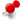# regex and to_number()ClassicListThreaded6 messagesOpen this post in threaded view
|

## regex and to_number()

 Consider this code: ```local x = 'aaabbb12345' local y = x.gsub('[ab]+', '') ```Then try to print the info like this: ```A = <%=Luan.to_number(y)%> B = <%=Luan.to_number(x.gsub('[ab]+', ''))%> ```The output is: ```A = 12345 B = nil ```Why?
Open this post in threaded view
|

## Re: regex and to_number()

 Administrator The problem is that gsub returns 2 values, the second value being the number of matches.  So you are calling to_number("12345",1) .  The second arg to to_number() is the base, and since this isn't valid, it returns nil. I followed Lua in having to_number() return a nil when there is an error, but maybe I should throw an error.  The error in this case would be "radix 1 less than Character.MIN_RADIX" (from java).  What do you think? Woe to those who call bad good and good bad -- Isaiah 5:20 Following the Old Testament, not evil modern culture
Open this post in threaded view
|

## Re: regex and to_number()

 Administrator Another option is to put to_number in the String module.  Then you would have: ```A = <%=y.to_number()%> B = <%=x.gsub('[ab]+', '').to_number()%> ```If you want to use a base, you could do: ```from hex = <%="1a".to_number(16)%> ``` Woe to those who call bad good and good bad -- Isaiah 5:20 Following the Old Testament, not evil modern culture
Open this post in threaded view
|

## Re: regex and to_number()

 fschmidt wrote Another option is to put to_number in the String module.  Then you would have: ```A = <%=y.to_number()%> B = <%=x.gsub('[ab]+', '').to_number()%> ```If you want to use a base, you could do: ```from hex = <%="1a".to_number(16)%> ``` I guess String to Number is 99.9% of the cases, so this suggestion is fine.